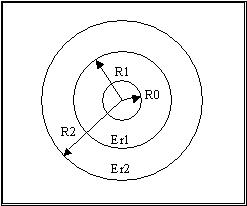Enter the Dimensions and Permittivity of the dielectric regions.Zo=SQRT(1000*L/C)

C=2*PI()*8.8541878*Er1*Er2/(Er1*LN(R2/R1)+Er2*LN(R1/R0))/3.2808

L=200*LN(R2/R0)/3.2808

Keff=Er1*Er2*LN(R2/R0)/(Er1*LN(R2/R1)+Er2*LN(R1/R0))

* Note: all dimensions are in units of your choice. Support The USA and use inches!